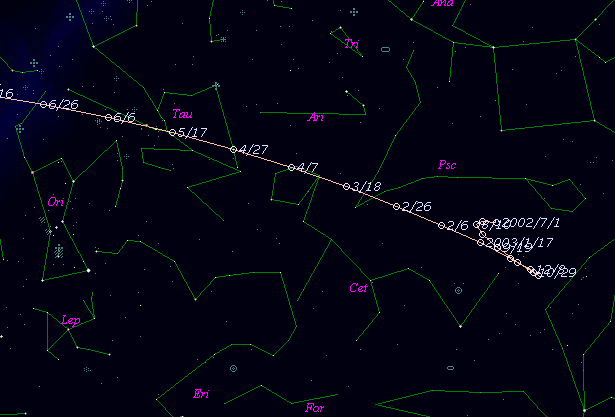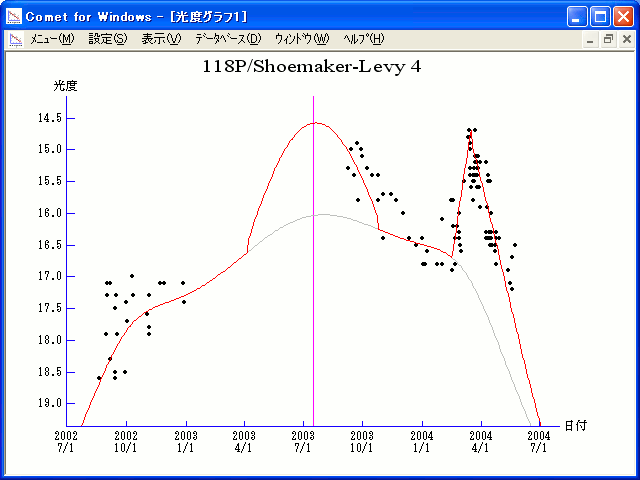# 118P/Shoemaker-Levy 4 (2003)###Pictures###Orbital Elements

```Epoch 2003 July 20.0 TT = JDT 2452840.5
T 2003 July 16.7602 TT                                  MPCN
q   2.011032             (2000.0)            P               Q
n   0.1517935      Peri.  302.0866      -0.0762946      -0.9946840
a   3.480438       Node   152.0394      +0.9573044      -0.0924708
e   0.422190       Incl.    8.4822      +0.2788323      +0.0453090
P   6.49
From 278 observations 1991-2002, mean residual 0".7.  Nongravitational
parameters A1 = +1.44, A2 = -0.1486.
```

###Finding Charts###Magnitudes Graph

```        m1 =  8.2 + 5 log d +  18.0 log r  [    ,-100]  (             - 2003 Apr.  7)
m1 = -6.0 + 5 log d +  60.0 log r  [-100, 100]  (2003 Apr.  7 - 2003 Oct. 24)
m1 =  8.2 + 5 log d +  18.0 log r  [ 100, 215]  (2003 Oct. 24 - 2004 Feb. 16)
m1 = 56.5 + 5 log d - 100   log r  [ 215, 245]  (2004 Feb. 16 - 2004 Mar. 17)
m1 = -5.6 + 5 log d +  45   log r  [ 245,    ]  (2004 Mar. 17 -             )

* Gray curve is:  m1 = 8.2 + 5 log d + 18.0 log r
```##### The orbital elements are published at the MPC Ephemerides and Orbital Elements. The charts are made with StellaNavigator Ver.2.0 for Windows (AstroArts / ASCII). The magnitudes graphs are made with Comet for Windows.